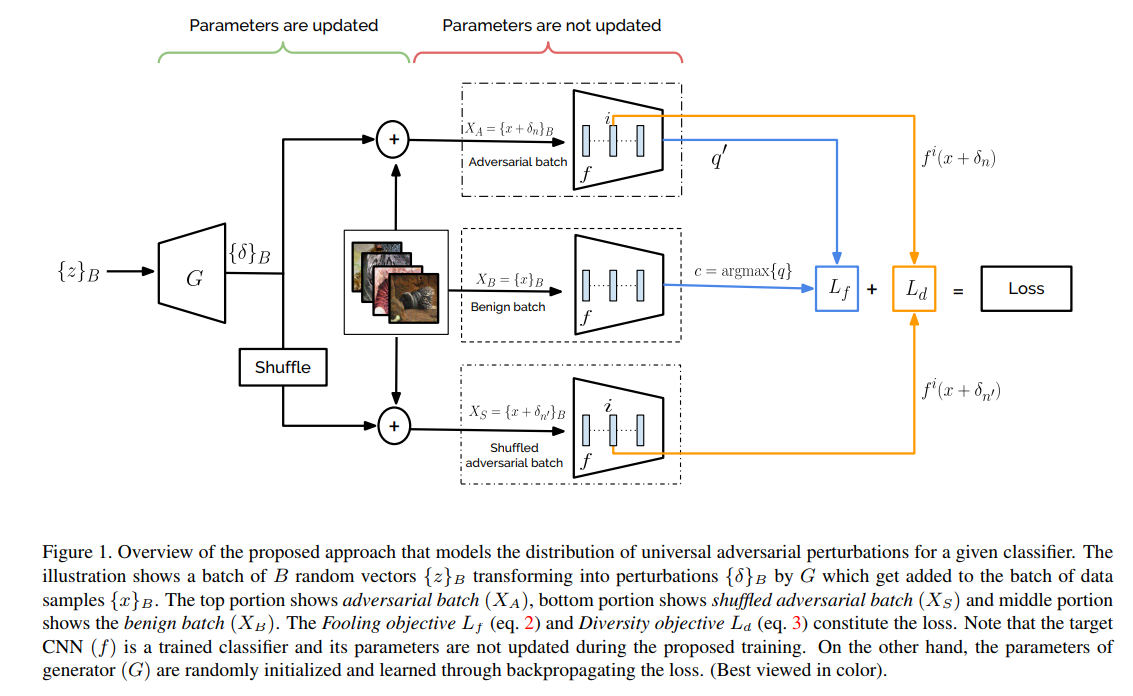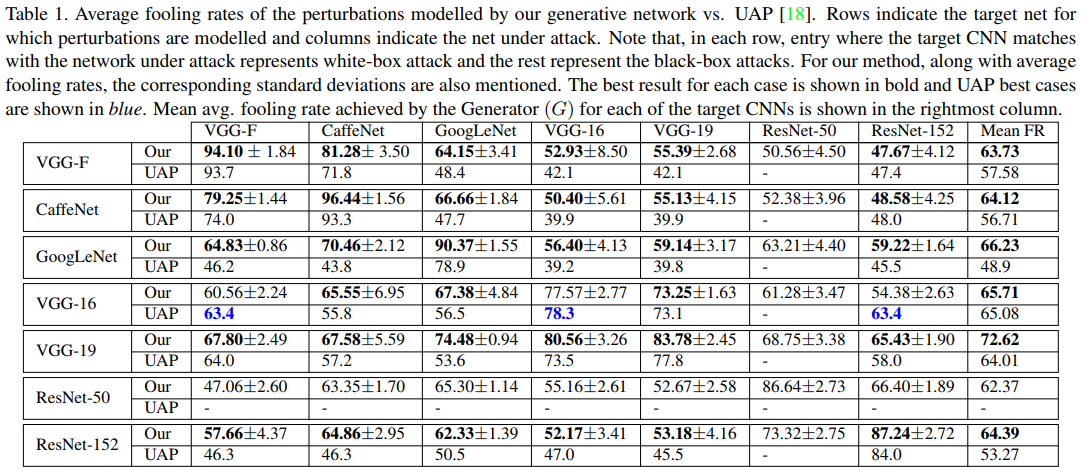The proposed method creates a universal adversarial noise generator. The goal is to generate noise that will fool most classifiers.### Methodology

• Select a model $f$ (Resnet, VGG, etc).
• Get the prediction for an image $$x$$.
• Generate a noise matrix $$\delta$$.
• Get the prediction for an augmented image $$x + \delta$$.

### Losses

• Fooling loss : $$L_{f} = - log(1 - f(x + \delta)_{c})$$ where $$c = argmax_l f(x)$$.
• Diversity loss done inside a batch of size $$B$$ and at a layer $$i$$ : $$L_{d} = - \sum_{n=1}^{B} d(f^i(x_n + \delta_n), f^i(x_n + \delta_{n'}))$$ where $$n' \neq n$$ and is choose randomly. This loss encourage diversity by comparing feature maps inside the classifier.

### Results